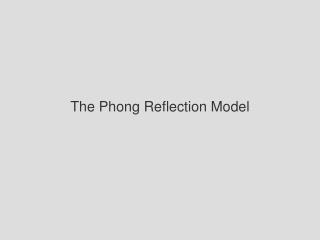DownloadDownload PresentationThe Phong Reflection Model

# The Phong Reflection Model

Download Presentation## The Phong Reflection Model

- - - - - - - - - - - - - - - - - - - - - - - - - - - E N D - - - - - - - - - - - - - - - - - - - - - - - - - - -
##### Presentation Transcript

1. The Phong Reflection Model

2. Lira Liga Liba Li = Lird Ligd Libd Lirs Ligs Libs Phong Reflection Model Supports three types of light-surface interactions - - ambient - diffuse - specular Each source has an ambient, a diffuse and a specular component for each of the three primary colors

3. Rira Riga Riba Ri = Rird Rigd Ribd Rirs Rigs Ribs Phong Reflection Model Supports three types of light-surface interactions - - ambient - diffuse - specular The amount of light reflected at each surface point has a has an ambient, a diffuse and a specular component for each of the three primary colors

4. We obtain the total intensity by adding the contributions of all sources and possibly a global ambient term Ir= ( Iira + Iird + Iirs ) + Iar i Phong Reflection Model We compute the contribution for each color source by adding the ambient, diffuse and specular components Lir= Rira Lira + Rird Lird + Rirs Lirs = Iira + Iird + Iirs

5. Phong Reflection Model n v l r p

6. pov: ambient 1.0 Ambient Reflection The same at every point on surface. Ra = kawhere 0 <= ka <= 1 kaindicates the percentage of ambient light reflected Ia = kaLa

7. 2 a + bd + cd we can add attenuation as a function of distance d Id = ---------------- (l n)Ld kd . pov: diffuse 1.0 Diffuse Reflection Diffuse reflections are from rough surfaces that scatter light in many directions. Also called Lambertian surfaces. Rd ~ cos(theta),where theta is the angle between n and l Id = kd(cos(theta))Ld ,where kd is the diffuse reflectivity coefficient Id = kd(l n)Ld,(assuming I & n are unit vectors) .

8. pov: specular 1.0 Specular Reflection Most reflected rays are close to the ideal reflection direction so a highlight (bright spot) appears on the surface. This occurs because the surfaces are smooth. The amount of light seen depends on the angle phi between r and v Is = ksLs cos (phi) , where a represents how tight the highlight is and 0 <= ks <= 1 Is = ksLs(r v) ,(assuming r & n are unit vectors) a . a

9. + = Id = ---------------- (kdLd(l n) + KsLs(r v) ) + kaLa 2 a + bd + cd . . 1 a Phong Reflection Model So finally the PHONG MODEL (for EACH COLOR and EACH LIGHT SOURCE) is ....

10. = Id = ---------------- (kdLd(l n) + KsLs(r v) ) + kaLa 2 a + bd + cd . . 1 a Phong Reflection Model So finally the PHONG MODEL (for EACH COLOR and EACH LIGHT SOURCE) is .... + +

11. Polygonal Shading To give a more realistic shading across the face of a flat polygon, possibly with differently calculated normals at each vertex, we want to vary the shading so the surface appears to curve properly between the vertices. Imagine a polygon on a sphere surface with vertex normals perpendicular to the sphere not to each polygon. We want it to look like a sphere.